Caffe
caffe::MultinomialLogisticLossLayer< Dtype > Class Template Reference

Computes the multinomial logistic loss for a one-of-many classification task, directly taking a predicted probability distribution as input. More...

#include <multinomial_logistic_loss_layer.hpp>

Inheritance diagram for caffe::MultinomialLogisticLossLayer< Dtype >: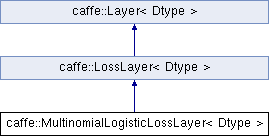## Public Member Functions

MultinomialLogisticLossLayer (const LayerParameter &param)

virtual void Reshape (const vector< Blob< Dtype > *> &bottom, const vector< Blob< Dtype > *> &top)
Adjust the shapes of top blobs and internal buffers to accommodate the shapes of the bottom blobs. More...

virtual const char * type () const
Returns the layer type.Public Member Functions inherited from caffe::LossLayer< Dtype >
LossLayer (const LayerParameter &param)

virtual void LayerSetUp (const vector< Blob< Dtype > *> &bottom, const vector< Blob< Dtype > *> &top)
Does layer-specific setup: your layer should implement this function as well as Reshape. More...

virtual int ExactNumBottomBlobs () const
Returns the exact number of bottom blobs required by the layer, or -1 if no exact number is required. More...

virtual bool AutoTopBlobs () const
For convenience and backwards compatibility, instruct the Net to automatically allocate a single top Blob for LossLayers, into which they output their singleton loss, (even if the user didn't specify one in the prototxt, etc.).

virtual int ExactNumTopBlobs () const
Returns the exact number of top blobs required by the layer, or -1 if no exact number is required. More...

virtual bool AllowForceBackward (const int bottom_index) constPublic Member Functions inherited from caffe::Layer< Dtype >
Layer (const LayerParameter &param)

void SetUp (const vector< Blob< Dtype > *> &bottom, const vector< Blob< Dtype > *> &top)
Implements common layer setup functionality. More...

Dtype Forward (const vector< Blob< Dtype > *> &bottom, const vector< Blob< Dtype > *> &top)
Given the bottom blobs, compute the top blobs and the loss. More...

void Backward (const vector< Blob< Dtype > *> &top, const vector< bool > &propagate_down, const vector< Blob< Dtype > *> &bottom)
Given the top blob error gradients, compute the bottom blob error gradients. More...

vector< shared_ptr< Blob< Dtype > > > & blobs ()
Returns the vector of learnable parameter blobs.

const LayerParameter & layer_param () const
Returns the layer parameter.

virtual void ToProto (LayerParameter *param, bool write_diff=false)
Writes the layer parameter to a protocol buffer.

Dtype loss (const int top_index) const
Returns the scalar loss associated with a top blob at a given index.

void set_loss (const int top_index, const Dtype value)
Sets the loss associated with a top blob at a given index.

virtual int MinBottomBlobs () const
Returns the minimum number of bottom blobs required by the layer, or -1 if no minimum number is required. More...

virtual int MaxBottomBlobs () const
Returns the maximum number of bottom blobs required by the layer, or -1 if no maximum number is required. More...

virtual int MinTopBlobs () const
Returns the minimum number of top blobs required by the layer, or -1 if no minimum number is required. More...

virtual int MaxTopBlobs () const
Returns the maximum number of top blobs required by the layer, or -1 if no maximum number is required. More...

virtual bool EqualNumBottomTopBlobs () const
Returns true if the layer requires an equal number of bottom and top blobs. More...

bool param_propagate_down (const int param_id)
Specifies whether the layer should compute gradients w.r.t. a parameter at a particular index given by param_id. More...

void set_param_propagate_down (const int param_id, const bool value)
Sets whether the layer should compute gradients w.r.t. a parameter at a particular index given by param_id.

## Protected Member Functions

virtual void Forward_cpu (const vector< Blob< Dtype > *> &bottom, const vector< Blob< Dtype > *> &top)
Computes the multinomial logistic loss for a one-of-many classification task, directly taking a predicted probability distribution as input. More...

virtual void Backward_cpu (const vector< Blob< Dtype > *> &top, const vector< bool > &propagate_down, const vector< Blob< Dtype > *> &bottom)
Computes the multinomial logistic loss error gradient w.r.t. the predictions. More...Protected Member Functions inherited from caffe::Layer< Dtype >
virtual void Forward_gpu (const vector< Blob< Dtype > *> &bottom, const vector< Blob< Dtype > *> &top)
Using the GPU device, compute the layer output. Fall back to Forward_cpu() if unavailable.

virtual void Backward_gpu (const vector< Blob< Dtype > *> &top, const vector< bool > &propagate_down, const vector< Blob< Dtype > *> &bottom)
Using the GPU device, compute the gradients for any parameters and for the bottom blobs if propagate_down is true. Fall back to Backward_cpu() if unavailable.

virtual void CheckBlobCounts (const vector< Blob< Dtype > *> &bottom, const vector< Blob< Dtype > *> &top)

void SetLossWeights (const vector< Blob< Dtype > *> &top)Protected Attributes inherited from caffe::Layer< Dtype >
LayerParameter layer_param_

Phase phase_

vector< shared_ptr< Blob< Dtype > > > blobs_

vector< bool > param_propagate_down_

vector< Dtype > loss_

## Detailed Description

### template<typename Dtype> class caffe::MultinomialLogisticLossLayer< Dtype >

Computes the multinomial logistic loss for a one-of-many classification task, directly taking a predicted probability distribution as input.

When predictions are not already a probability distribution, you should instead use the SoftmaxWithLossLayer, which maps predictions to a distribution using the SoftmaxLayer, before computing the multinomial logistic loss. The SoftmaxWithLossLayer should be preferred over separate SoftmaxLayer + MultinomialLogisticLossLayer as its gradient computation is more numerically stable.

Parameters
 bottom input Blob vector (length 2)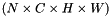the predictions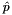, a Blob with values in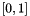indicating the predicted probability of each of the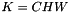classes. Each prediction vector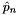should sum to 1 as in a probability distribution: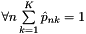.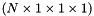the labels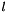, an integer-valued Blob with values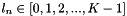indicating the correct class label among theclasses top output Blob vector (length 1)the computed multinomial logistic loss: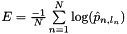## ◆ Backward_cpu()

template<typename Dtype >
 void caffe::MultinomialLogisticLossLayer< Dtype >::Backward_cpu ( const vector< Blob< Dtype > *> & top, const vector< bool > & propagate_down, const vector< Blob< Dtype > *> & bottom )
protectedvirtual

Computes the multinomial logistic loss error gradient w.r.t. the predictions.

Gradients cannot be computed with respect to the label inputs (bottom), so this method ignores bottom and requires !propagate_down, crashing if propagate_down is set.

Parameters
 top output Blob vector (length 1), providing the error gradient with respect to the outputsThis Blob's diff will simply contain the loss_weight*, asis the coefficient of this layer's outputin the overall Net loss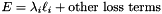; hence. (*Assuming that this top Blob is not used as a bottom (input) by any other layer of the Net.) propagate_down see Layer::Backward. propagate_down must be false as we can't compute gradients with respect to the labels. bottom input Blob vector (length 2)the predictions; Backward computes diff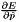the labels – ignored as we can't compute their error gradients

Implements caffe::Layer< Dtype >.

## ◆ Forward_cpu()

template<typename Dtype >
 void caffe::MultinomialLogisticLossLayer< Dtype >::Forward_cpu ( const vector< Blob< Dtype > *> & bottom, const vector< Blob< Dtype > *> & top )
protectedvirtual

Computes the multinomial logistic loss for a one-of-many classification task, directly taking a predicted probability distribution as input.

When predictions are not already a probability distribution, you should instead use the SoftmaxWithLossLayer, which maps predictions to a distribution using the SoftmaxLayer, before computing the multinomial logistic loss. The SoftmaxWithLossLayer should be preferred over separate SoftmaxLayer + MultinomialLogisticLossLayer as its gradient computation is more numerically stable.

Parameters
 bottom input Blob vector (length 2)the predictions, a Blob with values inindicating the predicted probability of each of theclasses. Each prediction vectorshould sum to 1 as in a probability distribution:.the labels, an integer-valued Blob with valuesindicating the correct class label among theclasses top output Blob vector (length 1)the computed multinomial logistic loss:Implements caffe::Layer< Dtype >.

## ◆ Reshape()

template<typename Dtype >
 void caffe::MultinomialLogisticLossLayer< Dtype >::Reshape ( const vector< Blob< Dtype > *> & bottom, const vector< Blob< Dtype > *> & top )
virtual

Adjust the shapes of top blobs and internal buffers to accommodate the shapes of the bottom blobs.

Parameters
 bottom the input blobs, with the requested input shapes top the top blobs, which should be reshaped as needed

This method should reshape top blobs as needed according to the shapes of the bottom (input) blobs, as well as reshaping any internal buffers and making any other necessary adjustments so that the layer can accommodate the bottom blobs.

Reimplemented from caffe::LossLayer< Dtype >.

The documentation for this class was generated from the following files: